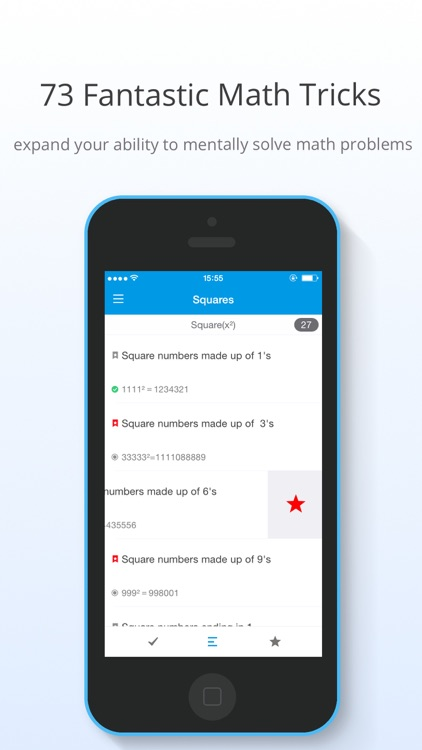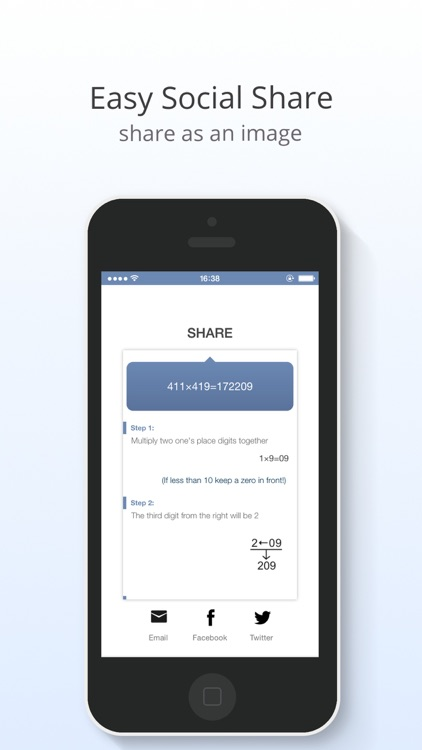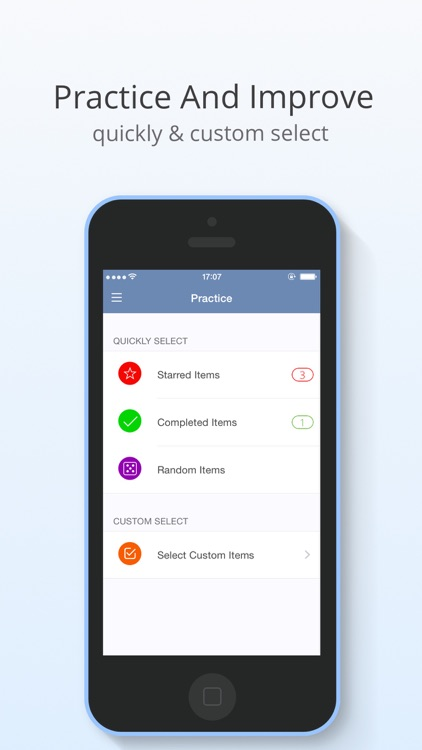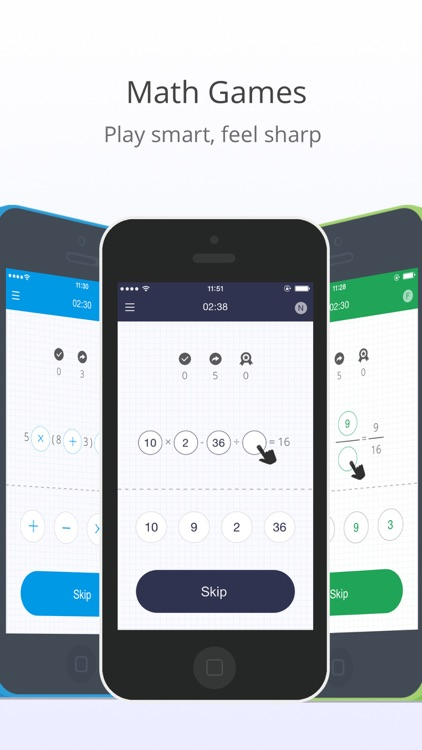# Mencal

by INSTANT.CJ\$1.99 in the App Store

Work on your mental math skills with this educational app.

Mencal is an app help you expand your ability to mentally solve math problems. All of us can do simple addition and subtraction of one or two digit numbers without a calculator.Now mencal is here to kick that ability into overdrive.### App Details

Version
3.70
Rating
(5)
Size
9Mb
Genre
Education Reference
Last updated
January 1, 2018
Release date
April 26, 2013

### Mencal is \$1.99 but there are more add-ons

• \$0.99

Unlock all math games

### App Screenshots### App Store Description

Mencal is an app help you expand your ability to mentally solve math problems. All of us can do simple addition and subtraction of one or two digit numbers without a calculator.Now mencal is here to kick that ability into overdrive.

Mencal provides you with lessons and simple tricks to allow you to: work with three digit numbers; fractions of different styles regardless if you are adding them or subtracting them; and finding sums with multiplication and division. Then it will test you on your grasp and knowledge of the methods you've learned.

• Example:
- Same ten's digit,1's sum to 10
38×32=1216
①:Multiply tens place digit by itself plus 1
(3+1)×3=12
②:Add two zeros to the end of step①
12<—00=1200
③:Multiply the two one's digits together
8×2=16
④:Add the results of step ②and③
1200+16=1216

• Quickly Square:
- Square numbers made up of 1's
- Square numbers made up of 3's
- Square numbers in the 90's
- Square numbers ten`s digit is 0
- Square a number ending in 25
- Square numbers made up of 6's
- Square numbers made up of 9's
- Square a number ending in 1
- Square a number ending in 5
- Square a number ending in 9
- Square numbers in the 10's
- Square numbers in the 40's
- Square numbers in the 50's
- Square numbers in the 500's
- Square numbers in the 600's
- Square numbers in the 700's
- Square numbers in the 800's
- Square numbers in the 900's

•Standard:
- Same ten's digit, 1's sum is 10
- Same ten's digit, 1's sum isn`t 10
- Same 1's digit, ten's digits sum is 10
- Same 1's digit, ten's digits sum isn`t 10
- two-digit number ab×cc (a+b=10)
- ab×ccc.....c (a+b=10)
- Multiply by 11
- 2-digit number if 1's digit is 1
- 2-digit number if tens place digit is 1
- 2-digit number ×2-digit number(universal)
- Multiply differing by 5
- Multiply differing by 6
- Multiply differing by 7
- Multiply differing by 8
- Multiply differing by 9
- Multiply differing by 10
- Multiply two numbers that in the 90‘s
- Two-digit number like ab+ba
- Three-digit number like abc+cba
- Two fractions like 1/a+1/b
- Two fractions like (a-1)/a + (b-1)/b
- Sum of first n odd numbers
- Sum of first n even numbers
- Two-digit number like ab-ba
- Three-digit number like abc-cba
- m-n if m+n=100,1000,10000...
- Two fractions like 1/a-1/b
- Two fractions like c/a-c/b
- Two fractions like (a-1)/a-(b-1)/b
- Divide any number by 5
- Divide any number by 25
- Divide any number by 125
- Divide any 3-figure number by 9

"This app rocks!! Great for Math Team practice!" -by Bubba Smith
"Great app, make me feel less stupid" -by Howie_Serious
"Its a useful app for anyone who wants to know the numbers deeper" -by sjzbim
"It's a good app for both kids and sometimes even adults" -by Willwang

Love mencal or have a question? We would love to hear your feedback! Contact us anytime at [email protected]

Disclaimer:
AppAdvice does not own this application and only provides images and links contained in the iTunes Search API, to help our users find the best apps to download. If you are the developer of this app and would like your information removed, please send a request to [email protected] and your information will be removed.